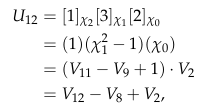# Non-induced modular representations of cyclic groups

### Liam Jolliffe and Robert A. SpencerAbstract: We compute the ring of non-induced representations for a cyclic group, $$C_n$$, over an arbitrary field and show that it has rank $$\varphi(n)$$, where $$\varphi$$ is Euler’s totient function - independent of the characteristic of the field. Along the way, we obtain a “pick-a-number” trick; expressing an integer $$n$$ as a sum of products of $$p$$-adic digits of related integers.

A draft of this work is available on the ArXiv.

#### Cite with BibTex

 @misc{jolliffe2021noninduced,
title={Non-induced modular representations of cyclic groups},
author={Liam Jolliffe and Robert A. Spencer},
year={2021},
eprint={2111.09187},
archivePrefix={arXiv},
primaryClass={math.RT}
}